### A Dark Secret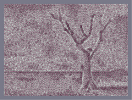Hover over the thumbnail for a full-size version.

Author mrgy05 author:mrgy05 bitesized dead desert n-art nonplayable rated secret 2007-08-20 2007-10-06 5 by 165 people. \$A Dark Secret#mrgy05#none#00000000000000000000000000000000000000000000000000000000000000000000000000000000000000000000000000000000000000000000000000000000000000000000000000000000000000000000000000000000000000000000000000000000000000000000000000000000000000000000000000000000000000000000000000000000000000000000000000000000000000000000000000000000000000000000000000000000000000000000000000000000000000000000000000000000000000000000000000000000000000000000000000000000000000000000000000000000000000000000000000000000000000000000000000000000000000000000000000000000000000000000000000000000000000000000000000000000000000000000000000000000000000000000000000000000000000000000000000000000000000000000000000000000000000000000000000000000000000000|3^518,531!3^521,523!3^524,517!3^527,509!3^529,501!3^511,537!3^513,526!3^516,517!3^518,505!3^501,536!3^492,536!3^484,541!3^494,530!3^501,526!3^501,515!3^509,516!3^510,505!3^501,502!3^517,494!3^509,494!3^529,489!3^591,535!3^587,535!3^582,535!3^574,535!3^569,535!3^563,535!3^557,535!3^557,531!3^556,526!3^552,521!3^587,529!3^580,529!3^574,529!3^568,527!3^562,522!3^557,517!3^577,522!3^574,521!3^570,519!3^565,514!3^562,510!3^575,514!3^571,508!3^566,502!3^561,496!3^558,491!3^556,483!3^561,506!3^554,501!3^551,509!3^543,501!3^547,512!3^542,504!3^533,492!3^541,489!3^550,492!3^547,483!3^554,476!3^544,473!3^539,480!3^535,485!3^531,478!3^542,471!3^554,465!3^543,461!3^537,468!3^547,453!3^543,445!3^542,433!3^540,421!3^539,412!3^539,404!3^539,396!3^524,485!3^513,483!3^502,490!3^504,477!3^524,473!3^517,476!3^510,471!3^531,467!3^523,463!3^533,458!3^539,451!3^533,446!3^533,437!3^534,428!3^533,421!3^531,414!3^531,403!3^525,451!3^525,444!3^523,432!3^526,422!3^522,410!3^517,466!3^515,456!3^516,446!3^520,437!3^520,424!3^506,464!3^507,455!3^506,444!3^509,435!3^503,429!3^500,420!3^496,415!3^488,407!3^492,402!3^493,395!3^510,429!3^510,420!3^517,417!3^506,410!3^499,403!3^503,397!3^512,404!3^524,400!3^516,395!3^509,390!3^540,385!3^534,389!3^528,393!3^522,387!3^514,382!3^508,378!3^509,370!3^507,362!3^505,351!3^505,342!3^533,382!3^524,382!3^519,373!3^517,364!3^516,353!3^516,347!3^541,372!3^539,363!3^537,395!3^537,405!3^538,414!3^540,427!3^531,403!3^535,408!3^541,439!3^534,445!3^529,433!3^532,421!3^531,460!3^548,460!3^531,372!3^527,366!3^533,357!3^525,354!3^534,349!3^527,344!3^519,337!3^510,334!3^503,332!3^504,322!3^513,325!3^526,327!3^521,329!3^531,338!3^535,330!3^541,354!3^547,345!3^551,335!3^554,326!3^557,318!3^565,312!3^572,303!3^578,297!3^587,289!3^591,282!3^593,268!3^601,256!3^604,243!3^607,244!3^607,234!3^605,227!3^608,216!3^610,201!3^616,192!3^619,182!3^614,177!3^609,167!3^608,161!3^607,152!3^603,146!3^603,138!3^603,133!3^603,125!3^604,118!3^604,109!3^605,99!3^606,95!3^609,93!3^611,89!3^624,177!3^628,174!3^631,170!3^631,165!3^631,161!3^635,154!3^642,149!3^649,141!3^654,134!3^655,121!3^663,108!3^661,125!3^670,123!3^621,165!3^621,156!3^615,152!3^613,146!3^617,187!3^559,308!3^568,297!3^574,285!3^579,277!3^585,266!3^590,254!3^596,242!3^599,231!3^596,220!3^596,207!3^597,201!3^602,192!3^604,191!3^607,185!3^542,345!3^546,338!3^552,330!3^558,322!3^567,307!3^574,297!3^584,287!3^590,275!3^593,266!3^595,256!3^603,246!3^602,236!3^603,219!3^607,208!3^607,196!3^611,188!3^609,227!3^609,221!3^609,211!3^609,203!3^609,192!3^613,245!3^614,240!3^617,241!3^597,133!3^592,128!3^537,323!3^534,323!3^527,319!3^541,314!3^533,313!3^548,306!3^547,300!3^543,303!3^531,304!3^523,314!3^537,296!3^545,292!3^537,291!3^523,305!3^517,315!3^511,315!3^504,313!3^515,305!3^508,300!3^502,293!3^496,287!3^506,308!3^498,302!3^493,297!3^497,308!3^488,301!3^480,297!3^472,294!3^466,288!3^485,290!3^475,283!3^482,283!3^465,277!3^458,273!3^463,280!3^462,272!3^463,263!3^465,268!3^473,266!3^471,259!3^463,257!3^456,255!3^459,249!3^457,251!3^453,245!3^458,240!3^449,246!3^446,239!3^452,236!3^448,229!3^443,235!3^438,227!3^443,223!3^438,221!3^429,217!3^435,214!3^422,212!3^408,194!3^408,189!3^405,181!3^397,175!3^406,189!3^399,183!3^394,181!3^388,180!3^396,177!3^391,175!3^387,170!3^382,165!3^379,158!3^379,152!3^375,146!3^374,138!3^371,134!3^367,130!3^363,125!3^365,121!3^366,115!3^366,107!3^366,101!3^362,92!3^361,87!3^358,81!3^354,75!3^355,68!3^356,60!3^356,55!3^356,51!3^359,121!3^352,117!3^347,112!3^342,113!3^336,111!3^332,110!3^329,109!3^323,107!3^319,102!3^319,92!3^313,88!3^313,86!3^302,81!3^296,81!3^308,82!3^416,206!3^414,198!3^430,208!3^423,203!3^417,194!3^457,228!3^451,220!3^443,211!3^436,198!3^431,190!3^428,186!3^438,200!3^432,194!3^429,190!3^424,184!3^420,177!3^416,174!3^415,177!3^413,166!3^409,161!3^407,155!3^406,150!3^401,144!3^399,137!3^394,134!3^441,188!3^438,181!3^433,175!3^437,166!3^445,198!3^452,214!3^475,275!3^483,275!3^491,284!3^491,275!3^488,266!3^489,258!3^492,254!3^496,251!3^479,264!3^479,257!3^470,254!3^481,248!3^488,245!3^496,240!3^504,234!3^511,230!3^500,245!3^503,237!3^510,233!3^506,238!3^508,241!3^517,236!3^523,231!3^530,224!3^536,218!3^538,211!3^528,210!3^521,220!3^511,210!3^507,203!3^500,197!3^498,192!3^497,190!3^496,186!3^494,181!3^492,178!3^490,173!3^488,168!3^486,164!3^487,160!3^487,159!3^504,205!3^518,215!3^536,198!3^541,196!3^536,188!3^542,183!3^537,176!3^541,165!3^547,173!3^548,161!3^544,159!3^549,168!3^549,160!3^548,152!3^547,147!3^547,137!3^547,128!3^548,122!3^551,116!3^551,151!3^551,145!3^551,134!3^552,126!3^552,120!3^553,106!3^557,102!3^559,98!3^560,96!3^560,90!3^567,88!3^570,87!3^563,86!3^553,97!3^552,100!3^549,98!3^545,181!3^540,201!3^525,294!3^480,403!3^482,393!3^492,407!3^497,412!3^494,398!3^499,406!3^490,391!3^493,393!3^488,430!3^476,429!3^465,430!3^451,430!3^441,430!3^429,429!3^416,429!3^407,432!3^391,430!3^380,430!3^368,429!3^352,429!3^343,431!3^330,431!3^315,429!3^303,432!3^290,430!3^280,430!3^266,432!3^252,432!3^236,429!3^227,431!3^213,431!3^200,428!3^190,432!3^178,430!3^162,429!3^154,432!3^142,432!3^129,432!3^120,431!3^107,431!3^97,433!3^87,430!3^74,429!3^53,436!3^48,434!3^33,433!3^30,451!3^40,442!3^41,451!3^36,463!3^30,471!3^39,474!3^47,464!3^50,444!3^65,441!3^59,455!3^59,473!3^67,466!3^72,474!3^86,472!3^82,468!3^76,440!3^91,442!3^489,439!3^472,441!3^460,439!3^444,441!3^428,441!3^419,440!3^403,439!3^386,439!3^370,441!3^355,440!3^339,437!3^313,437!3^295,437!3^277,440!3^265,441!3^248,441!3^230,437!3^204,438!3^188,435!3^173,435!3^321,440!3^329,441!3^492,438!3^488,433!3^480,437!3^479,432!3^469,440!3^471,433!3^459,441!3^458,431!3^452,438!3^443,431!3^437,438!3^432,432!3^423,441!3^423,432!3^409,440!3^406,431!3^397,444!3^397,434!3^390,444!3^388,432!3^378,441!3^376,432!3^365,441!3^363,433!3^354,437!3^351,431!3^346,440!3^337,432!3^332,441!3^322,431!3^318,440!3^307,430!3^306,440!3^294,434!3^296,439!3^280,431!3^285,438!3^272,433!3^271,442!3^260,433!3^257,441!3^244,431!3^238,441!3^231,434!3^226,443!3^218,434!3^217,441!3^209,433!3^206,443!3^195,432!3^195,440!3^182,434!3^181,440!3^169,432!3^168,437!3^159,433!3^159,437!3^148,432!3^150,439!3^137,431!3^138,436!3^127,430!3^127,433!3^119,430!3^116,432!3^102,429!3^93,429!3^83,429!3^76,429!3^483,442!3^554,430!3^557,436!3^567,432!3^571,440!3^575,429!3^581,436!3^589,431!3^601,429!3^613,428!3^561,452!3^558,446!3^572,449!3^585,450!3^589,443!3^602,450!3^604,440!3^617,449!3^633,443!3^485,461!3^491,449!3^485,451!3^475,450!3^477,466!3^487,475!3^473,473!3^463,477!3^450,478!3^458,471!3^466,482!3^467,475!3^473,481!3^480,472!3^486,470!3^488,465!3^489,460!3^490,455!3^489,445!3^491,431!3^481,458!3^556,440!3^563,453!3^563,445!3^569,453!3^583,454!3^595,452!3^610,451!3^624,450!3^631,449!3^644,441!3^653,431!3^641,429!3^625,429!3^619,432!3^613,440!3^599,442!3^596,437!3^575,445!3^574,439!3^556,433!3^564,430!3^574,429!3^584,429!3^590,429!3^597,429!3^579,440!3^587,440!3^586,453!3^599,448!3^609,434!3^617,446!3^627,440!3^631,433!3^634,448!3^646,435!3^636,439!3^622,437!3^633,429!3^653,430!3^646,431!3^574,491!3^569,482!3^566,472!3^564,464!3^574,463!3^577,472!3^582,464!3^586,498!3^585,490!3^582,482!3^594,488!3^611,488!3^595,481!3^592,474!3^593,461!3^605,471!3^607,465!3^615,476!3^621,466!3^618,461!3^631,459!3^646,461!3^688,464!3^680,463!3^667,462!3^655,461!3^655,470!3^645,471!3^635,471!3^624,475!3^621,487!3^633,483!3^639,491!3^646,483!3^652,492!3^657,480!3^666,474!3^678,473!3^692,475!3^685,482!3^672,484!3^696,486!3^707,485!3^704,478!3^703,469!3^712,469!3^725,467!3^719,478!3^721,488!3^730,486!3^729,477!3^738,464!3^750,463!3^740,474!3^743,486!3^754,486!3^753,476!3^760,464!3^765,475!3^764,488!3^766,451!3^758,450!3^747,452!3^729,454!3^716,455!3^712,460!3^701,458!3^690,454!3^678,453!3^664,453!3^650,452!3^643,452!3^656,446!3^661,437!3^666,432!3^677,428!3^685,424!3^692,418!3^701,416!3^711,414!3^721,416!3^729,415!3^738,415!3^763,431!3^755,424!3^744,418!3^762,441!3^753,434!3^746,430!3^736,424!3^725,424!3^712,427!3^700,424!3^690,430!3^681,434!3^672,442!3^685,442!3^692,435!3^702,433!3^717,434!3^726,434!3^737,435!3^750,441!3^739,444!3^740,452!3^729,445!3^716,444!3^707,448!3^707,439!3^696,445!3^761,436!3^757,429!3^744,421!3^731,419!3^733,429!3^747,431!3^723,417!3^711,420!3^701,416!3^690,421!3^676,426!3^667,431!3^662,433!3^648,441!3^647,449!3^650,452!3^660,455!3^664,449!3^674,451!3^667,445!3^677,436!3^686,445!3^653,444!3^693,454!3^701,441!3^720,453!3^706,454!3^726,446!3^720,438!3^706,429!3^745,442!3^704,419!3^733,451!3^752,448!3^768,444!3^732,440!3^761,450!3^425,448!3^419,453!3^423,450!3^415,448!3^384,450!3^374,445!3^363,448!3^344,446!3^315,444!3^300,443!3^274,449!3^256,448!3^233,448!3^221,446!3^370,449!3^371,454!3^378,451!3^356,447!3^352,455!3^340,444!3^328,455!3^324,446!3^401,452!3^436,446!3^765,519!3^766,524!3^762,531!3^759,524!3^756,520!3^756,530!3^752,537!3^751,528!3^746,523!3^746,519!3^745,529!3^744,536!3^737,530!3^738,521!3^735,520!3^735,532!3^729,537!3^729,530!3^727,523!3^721,520!3^721,531!3^721,535!3^711,525!3^708,518!3^713,518!3^699,518!3^692,527!3^684,537!3^682,526!3^691,519!3^680,519!3^680,529!3^673,535!3^674,525!3^672,519!3^668,529!3^663,536!3^663,526!3^663,520!3^661,529!3^654,537!3^655,522!3^651,521!3^651,533!3^645,531!3^643,522!3^639,533!3^632,536!3^633,526!3^630,522!3^616,532!3^614,522!3^636,530!3^627,528!3^619,520!3^614,531!3^609,526!3^603,537!3^604,530!3^597,526!3^593,522!3^606,521!3^587,512!3^580,501!3^603,508!3^572,478!3^580,466!3^592,484!3^567,470!3^613,478!3^602,465!3^638,478!3^633,468!3^659,487!3^661,464!3^680,475!3^692,465!3^698,483!3^710,474!3^732,466!3^723,480!3^747,485!3^750,469!3^770,479!3^599,496!3^616,498!3^619,509!3^632,502!3^636,513!3^648,505!3^664,497!3^663,509!3^675,512!3^677,497!3^684,511!3^693,499!3^706,508!3^708,493!3^721,503!3^729,514!3^734,495!3^743,506!3^757,512!3^753,496!3^767,501!3^765,536!3^764,545!3^767,558!3^756,548!3^749,542!3^750,549!3^739,544!3^729,543!3^766,565!3^755,554!3^755,563!3^747,569!3^742,563!3^743,554!3^730,554!3^722,544!3^709,536!3^702,531!3^695,538!3^680,539!3^726,554!3^706,543!3^714,545!3^735,570!3^724,559!3^710,556!3^714,550!3^717,564!3^734,564!3^724,569!3^709,569!3^698,570!3^691,561!3^701,561!3^698,549!3^692,545!3^689,556!3^681,553!3^680,549!3^671,546!3^671,555!3^679,564!3^666,565!3^659,570!3^653,559!3^659,558!3^662,548!3^650,549!3^644,545!3^644,553!3^630,550!3^625,543!3^616,545!3^605,545!3^647,568!3^638,557!3^624,555!3^611,552!3^583,552!3^597,543!3^592,553!3^642,573!3^634,568!3^622,566!3^614,570!3^601,567!3^607,562!3^580,566!3^576,569!3^579,573!3^579,575!3^561,575!3^553,572!3^547,572!3^537,572!3^519,575!3^516,571!3^501,572!3^500,574!3^494,575!3^489,569!3^482,571!3^481,574!3^478,572!3^475,566!3^472,566!3^459,572!3^456,566!3^454,563!3^447,570!3^445,572!3^440,568!3^436,567!3^433,577!3^433,568!3^433,560!3^422,561!3^421,570!3^419,575!3^425,568!3^421,562!3^408,577!3^408,571!3^405,569!3^386,573!3^381,570!3^378,565!3^373,556!3^375,574!3^371,576!3^368,568!3^368,565!3^364,570!3^362,576!3^356,565!3^352,571!3^349,575!3^345,572!3^345,571!3^337,579!3^332,581!3^309,567!3^300,569!3^298,576!3^298,574!3^306,576!3^311,580!3^311,575!3^271,565!3^272,568!3^278,570!3^291,570!3^285,574!3^270,574!3^256,576!3^264,564!3^257,571!3^249,575!3^246,569!3^231,576!3^224,582!3^237,567!3^226,573!3^216,567!3^205,573!3^203,568!3^203,560!3^187,575!3^187,566!3^194,573!3^192,564!3^177,569!3^175,577!3^172,564!3^163,571!3^154,582!3^156,560!3^150,569!3^148,571!3^144,565!3^141,572!3^131,567!3^128,556!3^123,569!3^120,572!3^113,557!3^112,555!3^112,565!3^103,570!3^96,572!3^93,562!3^92,560!3^86,566!3^83,568!3^87,554!3^76,562!3^67,569!3^62,564!3^58,561!3^52,567!3^43,572!3^42,565!3^39,557!3^33,559!3^32,566!3^30,572!3^129,573!3^99,575!3^86,573!3^73,572!3^63,572!3^216,573!3^266,575!3^453,579!3^474,587!3^469,575!3^528,571!3^758,575!3^683,573!3^601,575!3^588,572!3^589,562!3^628,578!3^575,558!3^561,558!3^565,560!3^569,567!3^551,563!3^538,562!3^525,562!3^515,562!3^545,540!3^538,538!3^534,534!3^532,530!3^535,527!3^543,530!3^537,518!3^564,549!3^554,550!3^576,548!3^541,553!3^532,549!3^529,546!3^527,546!3^528,553!3^517,552!3^514,546!3^504,558!3^494,561!3^495,548!3^505,549!3^486,551!3^482,562!3^473,552!3^461,551!3^466,559!3^447,552!3^470,538!3^477,528!3^483,516!3^487,504!3^487,496!3^487,492!3^488,489!3^487,497!3^480,502!3^480,492!3^473,498!3^469,507!3^464,497!3^471,492!3^459,507!3^457,488!3^453,500!3^448,506!3^449,490!3^440,494!3^440,503!3^438,485!3^440,472!3^429,474!3^443,472!3^438,476!3^448,472!3^442,464!3^431,463!3^466,466!3^449,466!3^440,454!3^449,448!3^452,456!3^461,450!3^466,458!3^483,481!3^488,481!3^475,485!3^459,486!3^465,489!3^473,515!3^466,527!3^458,516!3^463,520!3^450,525!3^443,513!3^440,522!3^426,519!3^427,532!3^417,524!3^425,526!3^420,524!3^441,534!3^426,541!3^416,536!3^436,551!3^439,544!3^452,543!3^458,534!3^462,542!3^443,559!3^426,551!3^414,544!3^415,552!3^411,562!3^398,558!3^395,571!3^387,562!3^383,550!3^404,551!3^394,550!3^401,542!3^393,542!3^406,534!3^404,521!3^395,530!3^417,512!3^429,509!3^427,499!3^396,570!3^388,565!3^404,562!3^388,553!3^403,544!3^410,551!3^430,550!3^412,543!3^373,540!3^383,536!3^385,524!3^366,550!3^355,555!3^351,544!3^341,558!3^336,548!3^332,559!3^327,571!3^319,559!3^334,569!3^324,551!3^304,553!3^297,558!3^286,562!3^272,557!3^256,556!3^244,559!3^234,557!3^245,548!3^258,547!3^275,547!3^290,551!3^297,544!3^315,550!3^310,543!3^325,542!3^339,538!3^362,539!3^355,532!3^371,531!3^373,520!3^393,517!3^385,510!3^403,508!3^412,502!3^428,486!3^420,492!3^417,479!3^407,489!3^399,496!3^393,506!3^388,497!3^396,484!3^406,479!3^419,468!3^411,461!3^403,469!3^392,460!3^394,471!3^382,461!3^382,470!3^385,482!3^377,491!3^375,501!3^374,511!3^362,522!3^344,529!3^350,520!3^362,508!3^365,497!3^374,482!3^364,484!3^371,472!3^362,461!3^372,462!3^349,478!3^339,474!3^343,476!3^351,466!3^347,471!3^357,473!3^355,485!3^344,483!3^353,495!3^343,495!3^352,505!3^343,506!3^336,517!3^331,526!3^326,532!3^314,535!3^305,533!3^286,541!3^267,540!3^276,533!3^292,533!3^307,522!3^319,522!3^326,512!3^331,506!3^334,495!3^334,486!3^340,456!3^337,464!3^325,465!3^329,472!3^325,480!3^324,492!3^321,502!3^313,513!3^296,522!3^302,511!3^310,501!3^316,488!3^316,472!3^317,456!3^307,454!3^291,449!3^284,450!3^275,454!3^267,451!3^299,460!3^308,466!3^303,473!3^289,460!3^293,471!3^284,465!3^307,478!3^305,488!3^295,481!3^299,497!3^294,491!3^292,507!3^286,526!3^290,519!3^348,560!3^323,564!3^320,572!3^305,560!3^290,561!3^366,556!3^349,558!3^329,475!3^317,473!3^314,461!3^328,459!3^347,461!3^364,466!3^385,466!3^284,476!3^275,468!3^266,463!3^263,471!3^271,477!3^281,485!3^283,496!3^281,510!3^277,523!3^250,537!3^259,533!3^265,523!3^271,514!3^273,503!3^271,490!3^260,484!3^253,476!3^253,465!3^246,456!3^259,457!3^236,459!3^219,451!3^242,465!3^225,458!3^217,472!3^227,465!3^238,474!3^228,475!3^244,484!3^253,489!3^262,496!3^261,506!3^255,515!3^251,524!3^242,531!3^236,542!3^224,559!3^211,558!3^229,546!3^219,550!3^222,540!3^231,533!3^239,522!3^245,514!3^250,501!3^244,497!3^233,488!3^240,505!3^232,497!3^232,514!3^226,526!3^218,533!3^216,517!3^223,507!3^220,494!3^213,500!3^227,483!3^214,482!3^213,464!3^208,457!3^194,448!3^209,450!3^199,465!3^195,457!3^185,456!3^184,449!3^173,449!3^163,447!3^208,475!3^205,488!3^195,476!3^189,469!3^173,458!3^154,450!3^142,445!3^127,443!3^113,441!3^102,439!3^110,436!3^121,438!3^139,441!3^149,445!3^73,454!3^82,447!3^84,456!3^93,453!3^94,462!3^93,470!3^100,461!3^101,469!3^104,476!3^110,471!3^113,462!3^117,473!3^114,476!3^123,471!3^125,465!3^126,475!3^131,482!3^133,473!3^137,465!3^137,471!3^140,477!3^146,470!3^152,465!3^150,472!3^151,481!3^155,471!3^162,468!3^164,475!3^165,482!3^170,473!3^175,465!3^178,478!3^178,482!3^179,472!3^190,466!3^190,474!3^193,484!3^198,473!3^208,472!3^189,479!3^206,511!3^201,500!3^208,524!3^206,534!3^209,541!3^201,549!3^186,555!3^176,555!3^164,554!3^193,543!3^183,543!3^174,544!3^160,543!3^151,546!3^145,555!3^137,555!3^139,547!3^128,546!3^115,545!3^103,548!3^101,558!3^96,553!3^95,545!3^82,551!3^85,542!3^72,552!3^64,555!3^62,547!3^51,552!3^52,543!3^43,544!3^32,549!3^30,539!3^36,537!3^29,529!3^49,537!3^42,530!3^32,517!3^40,521!3^40,508!3^29,507!3^37,498!3^30,495!3^29,483!3^35,491!3^43,481!3^47,498!3^68,536!3^58,537!3^56,526!3^48,516!3^60,509!3^54,505!3^60,493!3^50,486!3^51,475!3^65,484!3^77,480!3^74,498!3^63,429!3^52,429!3^38,430!3^30,430!3^28,441!3^44,428!3^66,431!3^69,446!3^55,443!3^47,454!3^57,465!3^28,462!3^104,446!3^101,452!3^111,451!3^120,457!3^120,449!3^132,459!3^132,450!3^144,458!3^162,457!3^146,538!3^132,538!3^154,534!3^167,534!3^178,534!3^194,534!3^196,522!3^186,527!3^194,515!3^184,516!3^176,524!3^165,524!3^173,510!3^194,507!3^184,505!3^194,497!3^183,490!3^172,498!3^167,485!3^160,491!3^164,504!3^164,513!3^155,520!3^154,524!3^143,529!3^134,528!3^123,529!3^112,535!3^101,538!3^90,530!3^78,533!3^72,525!3^64,522!3^70,511!3^80,517!3^67,499!3^72,490!3^107,526!3^95,518!3^86,508!3^79,502!3^82,488!3^90,482!3^91,498!3^108,515!3^100,508!3^120,519!3^130,519!3^136,519!3^148,512!3^156,508!3^155,499!3^150,491!3^143,505!3^130,508!3^139,487!3^136,495!3^117,500!3^117,509!3^123,495!3^121,483!3^113,486!3^110,502!3^104,494!3^100,486!3^725,462!3^676,462!3^645,457!3^635,456!3^610,459!3^575,458!3^277,417!3^264,416!3^248,414!3^234,413!3^220,413!3^207,412!3^194,412!3^181,410!3^168,408!3^148,406!3^159,394!3^172,396!3^185,387!3^186,397!3^200,401!3^198,390!3^210,400!3^215,388!3^223,402!3^239,403!3^231,390!3^247,392!3^257,401!3^268,390!3^289,388!3^281,393!3^299,397!3^307,385!3^316,391!3^332,385!3^342,390!3^324,402!3^312,409!3^329,416!3^295,415!3^359,413!3^346,402!3^359,403!3^353,392!3^347,381!3^361,381!3^367,393!3^368,408!3^380,413!3^380,402!3^379,390!3^377,379!3^394,409!3^394,398!3^393,386!3^406,407!3^403,397!3^421,411!3^416,395!3^402,417!3^391,419!3^369,417!3^415,419!3^439,418!3^456,406!3^435,406!3^427,398!3^443,396!3^427,384!3^411,383!3^441,383!3^435,370!3^448,375!3^462,373!3^452,364!3^475,361!3^465,362!3^533,327!3^523,320!3^520,316!3^516,312!3^509,307!3^505,305!3^493,294!3^490,251!3^493,250!3^493,248!3^496,245!3^499,241!3^503,239!3^488,259!3^467,258!3^463,253!3^457,251!3^453,246!3^451,240!3^448,234!3^535,352!3^535,348!3^534,340!3^533,336!3^533,329!3^535,366!3^535,358!3^525,345!3^524,332!3^529,363!3^536,375!3^441,359!3^431,351!3^453,352!3^444,343!3^464,350!3^478,349!3^495,368!3^493,356!3^486,362!3^474,374!3^488,378!3^460,386!3^488,419!3^478,416!3^459,421!3^551,403!3^552,386!3^562,396!3^566,410!3^553,415!3^579,418!3^578,400!3^591,408!3^594,395!3^585,386!3^570,384!3^560,370!3^578,368!3^565,356!3^582,354!3^596,381!3^612,392!3^605,400!3^623,394!3^638,387!3^656,390!3^681,410!3^673,405!3^670,417!3^660,411!3^655,419!3^647,408!3^645,419!3^634,416!3^620,418!3^608,416!3^633,396!3^645,375!3^628,383!3^610,380!3^596,365!3^619,373!3^609,362!3^604,350!3^634,368!3^625,360!3^650,362!3^640,358!3^665,352!3^660,371!3^681,379!3^653,347!3^636,345!3^617,343!3^618,327!3^656,335!3^644,337!3^668,337!3^658,325!3^643,327!3^670,323!3^657,310!3^649,316!3^633,316!3^632,333!3^671,312!3^650,289!3^648,302!3^665,297!3^637,303!3^639,290!3^624,301!3^608,311!3^611,295!3^628,289!3^633,278!3^597,300!3^600,287!3^621,313!3^584,301!3^579,311!3^596,310!3^589,325!3^573,325!3^552,357!3^559,286!3^567,274!3^571,261!3^557,273!3^561,264!3^550,280!3^544,272!3^552,263!3^533,275!3^536,265!3^520,279!3^508,283!3^504,273!3^501,260!3^513,269!3^514,257!3^525,265!3^526,254!3^531,245!3^539,253!3^553,253!3^545,240!3^552,232!3^558,244!3^571,248!3^580,236!3^568,232!3^581,224!3^569,224!3^559,223!3^550,223!3^557,209!3^583,216!3^573,214!3^567,213!3^579,205!3^568,204!3^562,197!3^580,194!3^572,190!3^564,183!3^587,179!3^601,180!3^589,188!3^555,190!3^550,203!3^541,233!3^577,244!3^616,282!3^624,264!3^648,269!3^639,254!3^666,277!3^697,309!3^684,299!3^685,283!3^682,315!3^677,331!3^677,351!3^693,337!3^695,323!3^712,331!3^707,346!3^759,356!3^750,358!3^740,365!3^724,357!3^719,363!3^706,361!3^763,392!3^759,381!3^754,391!3^742,382!3^751,403!3^741,407!3^742,393!3^730,403!3^729,391!3^720,402!3^707,404!3^711,385!3^760,348!3^749,347!3^735,355!3^727,346!3^741,346!3^725,336!3^740,337!3^753,337!3^761,337!3^761,323!3^752,328!3^739,325!3^727,324!3^717,318!3^764,313!3^751,314!3^740,316!3^726,309!3^758,301!3^745,302!3^758,291!3^744,285!3^756,281!3^765,272!3^758,269!3^751,275!3^741,270!3^751,266!3^759,261!3^764,251!3^752,255!3^742,257!3^756,241!3^764,235!3^750,248!3^741,239!3^731,264!3^724,252!3^729,278!3^733,250!3^736,291!3^717,286!3^711,300!3^698,292!3^702,275!3^691,285!3^706,285!3^691,272!3^679,272!3^680,261!3^670,265!3^692,264!3^718,275!3^709,268!3^719,261!3^704,260!3^712,252!3^706,242!3^701,247!3^693,241!3^691,245!3^692,255!3^679,245!3^685,236!3^713,242!3^725,242!3^716,233!3^703,232!3^693,230!3^732,233!3^751,227!3^731,222!3^715,222!3^699,220!3^685,219!3^663,250!3^653,257!3^666,237!3^680,229!3^667,225!3^655,230!3^649,241!3^641,230!3^658,217!3^674,216!3^647,220!3^633,215!3^646,205!3^628,223!3^626,209!3^635,201!3^632,185!3^643,187!3^643,174!3^654,159!3^624,197!3^619,228!3^620,214!3^630,241!3^608,267!3^764,224!3^756,216!3^741,216!3^730,204!3^724,211!3^712,209!3^699,211!3^686,209!3^675,207!3^661,206!3^657,195!3^657,182!3^668,194!3^673,184!3^682,198!3^697,199!3^707,198!3^721,201!3^720,191!3^707,186!3^693,187!3^685,185!3^684,177!3^671,173!3^657,170!3^663,160!3^662,145!3^680,163!3^674,154!3^673,142!3^680,131!3^665,124!3^658,129!3^650,138!3^645,146!3^636,152!3^633,159!3^659,115!3^516,226!3^522,223!3^529,216!3^537,207!3^540,197!3^543,188!3^546,180!3^546,172!3^550,300!3^548,296!3^545,293!3^541,292!3^536,292!3^540,300!3^531,296!3^547,349!3^549,340!3^550,338!3^551,335!3^552,330!3^554,326!3^558,323!3^568,312!3^405,186!3^401,183!3^396,180!3^390,179!3^385,179!3^385,179!3^388,172!3^518,525!3^518,519!3^521,514!3^523,509!3^527,504!3^528,500!3^529,494!3^520,520!3^511,529!3^514,521!3^517,509!3^520,497!3^512,521!3^748,205!3^762,203!3^753,195!3^739,193!3^763,185!3^752,184!3^739,182!3^723,180!3^705,174!3^697,174!3^692,164!3^685,150!3^691,139!3^717,170!3^708,165!3^700,157!3^698,145!3^748,171!3^736,170!3^733,159!3^727,165!3^714,157!3^709,142!3^723,150!3^749,156!3^760,173!3^761,164!3^762,152!3^752,146!3^738,148!3^727,138!3^714,131!3^701,131!3^693,124!3^704,120!3^719,121!3^730,126!3^743,149!3^764,137!3^742,137!3^752,131!3^765,125!3^742,122!3^756,120!3^767,112!3^764,101!3^756,109!3^745,109!3^732,113!3^720,111!3^709,110!3^698,113!3^681,116!3^684,105!3^697,99!3^712,102!3^714,91!3^724,103!3^730,93!3^734,99!3^751,97!3^742,89!3^758,90!3^753,82!3^765,80!3^764,70!3^764,51!3^748,45!3^761,33!3^728,56!3^720,38!3^716,47!3^701,46!3^710,39!3^706,56!3^697,33!3^730,30!3^712,30!3^694,57!3^686,46!3^677,53!3^647,43!3^670,39!3^689,71!3^683,59!3^706,69!3^691,82!3^665,73!3^676,77!3^672,66!3^668,89!3^659,84!3^646,100!3^625,142!3^633,137!3^644,128!3^633,123!3^615,130!3^626,128!3^620,116!3^641,114!3^630,106!3^621,101!3^630,92!3^649,105!3^649,86!3^639,92!3^639,79!3^652,73!3^664,53!3^659,63!3^650,54!3^649,63!3^640,57!3^637,66!3^623,37!3^632,45!3^619,48!3^626,56!3^627,80!3^619,68!3^605,75!3^616,79!3^600,86!3^588,88!3^574,177!3^557,174!3^569,169!3^564,163!3^599,169!3^589,169!3^579,164!3^574,156!3^563,149!3^592,156!3^583,152!3^574,143!3^563,135!3^591,143!3^581,135!3^567,125!3^578,113!3^581,122!3^592,104!3^576,99!3^591,71!3^570,72!3^551,79!3^545,87!3^539,94!3^539,105!3^537,118!3^536,132!3^527,126!3^527,110!3^528,98!3^530,86!3^540,76!3^557,66!3^580,60!3^603,62!3^594,59!3^614,57!3^608,51!3^594,49!3^583,49!3^607,38!3^596,40!3^581,37!3^591,27!3^603,28!3^635,31!3^656,29!3^573,28!3^570,39!3^557,32!3^566,49!3^555,41!3^570,61!3^550,54!3^533,62!3^544,27!3^542,38!3^532,33!3^531,44!3^514,30!3^513,49!3^523,37!3^510,38!3^499,45!3^505,27!3^494,35!3^488,27!3^485,36!3^474,32!3^469,26!3^458,35!3^450,28!3^443,39!3^432,28!3^425,36!3^440,30!3^451,38!3^467,40!3^463,30!3^488,46!3^478,43!3^473,48!3^473,37!3^461,48!3^459,43!3^452,55!3^446,46!3^434,39!3^427,47!3^442,56!3^437,48!3^429,58!3^419,55!3^462,59!3^471,54!3^480,56!3^492,54!3^505,54!3^515,53!3^529,46!3^524,56!3^525,72!3^518,64!3^519,82!3^511,71!3^507,65!3^497,64!3^484,65!3^473,65!3^455,70!3^444,81!3^435,69!3^443,67!3^420,68!3^402,79!3^434,91!3^428,84!3^415,86!3^444,85!3^457,87!3^463,77!3^474,82!3^489,85!3^487,75!3^498,76!3^510,85!3^502,86!3^519,92!3^517,103!3^507,96!3^495,96!3^519,117!3^511,110!3^502,105!3^495,109!3^504,117!3^511,123!3^519,131!3^532,144!3^530,154!3^528,160!3^527,175!3^525,186!3^521,199!3^515,190!3^507,180!3^501,166!3^514,175!3^513,167!3^520,155!3^509,157!3^500,151!3^510,142!3^519,143!3^506,132!3^497,120!3^497,131!3^487,125!3^493,140!3^485,147!3^480,135!3^476,145!3^468,137!3^500,218!3^494,209!3^490,217!3^482,207!3^485,200!3^474,199!3^483,189!3^474,188!3^479,179!3^473,177!3^474,166!3^463,167!3^474,153!3^464,156!3^463,148!3^450,146!3^451,155!3^491,229!3^473,237!3^481,226!3^472,222!3^474,212!3^461,208!3^460,195!3^465,181!3^452,176!3^439,148!3^426,152!3^425,137!3^437,134!3^456,137!3^449,122!3^467,123!3^483,115!3^481,93!3^473,104!3^485,103!3^466,94!3^459,108!3^446,97!3^443,108!3^434,123!3^429,103!3^420,98!3^420,113!3^413,127!3^406,106!3^402,94!3^397,120!3^392,108!3^397,160!3^388,147!3^380,132!3^379,116!3^382,100!3^393,93!3^377,80!3^413,141!3^414,77!3^406,55!3^408,64!3^399,71!3^387,80!3^378,68!3^368,71!3^385,65!3^377,55!3^371,60!3^387,54!3^396,59!3^394,48!3^419,43!3^407,42!3^415,31!3^402,33!3^393,31!3^395,42!3^382,40!3^375,48!3^365,48!3^373,39!3^361,37!3^378,29!3^369,29!3^355,28!3^349,41!3^338,30!3^340,46!3^335,40!3^322,42!3^321,32!3^330,33!3^341,58!3^327,51!3^328,62!3^314,59!3^340,68!3^352,100!3^345,88!3^338,95!3^329,84!3^337,79!3^325,71!3^313,70!3^298,68!3^302,58!3^311,48!3^306,32!3^301,41!3^293,30!3^278,30!3^264,30!3^251,30!3^237,29!3^239,39!3^253,41!3^263,40!3^277,35!3^290,40!3^294,50!3^283,46!3^270,46!3^255,53!3^244,51!3^267,57!3^279,58!3^288,58!3^288,66!3^275,71!3^267,67!3^253,64!3^244,63!3^245,76!3^257,75!3^270,79!3^282,80!3^304,95!3^290,94!3^276,92!3^265,84!3^252,86!3^263,94!3^241,96!3^253,107!3^237,83!3^225,86!3^236,74!3^224,74!3^214,81!3^232,63!3^209,55!3^223,53!3^219,63!3^233,52!3^228,43!3^265,102!3^273,101!3^285,102!3^298,104!3^308,105!3^301,117!3^286,113!3^275,112!3^259,114!3^298,130!3^284,120!3^275,127!3^265,121!3^261,131!3^251,128!3^248,118!3^237,107!3^227,98!3^223,108!3^235,119!3^251,96!3^215,95!3^204,90!3^203,72!3^211,70!3^205,64!3^190,64!3^193,76!3^195,54!3^206,43!3^217,44!3^226,31!3^212,33!3^194,28!3^195,40!3^180,32!3^183,42!3^182,55!3^173,49!3^170,36!3^157,28!3^160,44!3^149,32!3^137,29!3^143,36!3^147,51!3^131,44!3^122,33!3^114,44!3^107,30!3^126,53!3^141,63!3^154,54!3^168,61!3^181,72!3^169,72!3^153,64!3^188,86!3^176,81!3^162,78!3^144,76!3^202,102!3^191,97!3^176,98!3^166,91!3^150,90!3^210,111!3^220,122!3^201,122!3^192,111!3^177,110!3^159,103!3^141,103!3^133,88!3^131,73!3^116,61!3^101,49!3^118,72!3^110,91!3^118,86!3^124,106!3^104,78!3^100,64!3^88,74!3^87,58!3^77,60!3^94,36!3^84,42!3^77,30!3^73,39!3^55,32!3^57,49!3^63,58!3^57,75!3^46,62!3^70,81!3^64,94!3^88,91!3^54,84!3^40,84!3^38,70!3^25,69!3^29,51!3^40,50!3^41,36!3^29,30!3^63,20!3^88,24!3^172,23!3^309,124!3^313,118!3^327,120!3^339,126!3^350,133!3^359,146!3^348,142!3^336,136!3^322,129!3^367,166!3^364,155!3^356,157!3^345,152!3^334,145!3^323,142!3^373,177!3^360,178!3^356,168!3^346,164!3^332,160!3^321,150!3^311,140!3^304,144!3^287,136!3^281,144!3^372,190!3^380,197!3^386,196!3^399,203!3^387,210!3^374,206!3^363,197!3^356,188!3^339,186!3^346,176!3^334,175!3^323,169!3^313,159!3^299,156!3^291,146!3^288,156!3^271,138!3^266,147!3^274,157!3^251,142!3^237,133!3^222,138!3^202,144!3^237,146!3^400,215!3^477,123!3^459,118!3^454,97!3^410,117!3^372,91!3^342,100!3^329,96!3^322,104!3^319,97!3^317,92!3^412,223!3^424,230!3^430,243!3^439,256!3^446,268!3^447,285!3^437,279!3^433,267!3^424,268!3^428,257!3^414,256!3^416,245!3^411,236!3^404,243!3^399,229!3^390,235!3^390,224!3^377,228!3^376,217!3^367,220!3^364,210!3^354,212!3^353,202!3^339,201!3^325,191!3^322,184!3^310,180!3^310,171!3^296,171!3^295,182!3^310,198!3^330,205!3^337,216!3^346,228!3^355,227!3^364,244!3^372,236!3^381,249!3^394,247!3^398,259!3^408,268!3^400,279!3^418,282!3^425,296!3^438,297!3^450,306!3^457,294!3^437,307!3^425,308!3^423,322!3^435,318!3^449,320!3^431,340!3^440,330!3^450,333!3^458,341!3^471,337!3^464,328!3^463,317!3^465,309!3^474,325!3^488,339!3^493,329!3^489,317!3^476,310!3^481,329!3^418,375!3^401,375!3^393,374!3^391,355!3^375,366!3^407,362!3^421,350!3^407,345!3^405,337!3^387,331!3^415,337!3^409,326!3^397,328!3^394,342!3^409,316!3^396,316!3^385,319!3^409,301!3^397,301!3^384,300!3^371,315!3^372,328!3^359,320!3^360,305!3^371,303!3^373,290!3^348,306!3^366,353!3^354,367!3^333,373!3^315,375!3^342,415!3^285,405!3^340,359!3^326,359!3^312,356!3^299,358!3^296,370!3^284,364!3^273,379!3^269,362!3^260,378!3^248,372!3^239,378!3^229,373!3^218,379!3^204,375!3^255,363!3^244,361!3^235,368!3^225,360!3^215,365!3^240,353!3^262,355!3^281,356!3^272,345!3^253,348!3^290,350!3^294,340!3^301,347!3^309,341!3^314,348!3^325,338!3^327,346!3^340,342!3^351,352!3^377,355!3^384,337!3^378,343!3^371,338!3^361,343!3^353,336!3^360,332!3^348,328!3^336,332!3^346,318!3^336,322!3^326,329!3^313,334!3^309,322!3^302,332!3^280,340!3^291,324!3^322,316!3^331,312!3^319,300!3^307,307!3^338,299!3^351,288!3^358,295!3^394,291!3^408,290!3^385,280!3^394,271!3^387,263!3^374,271!3^363,276!3^366,260!3^353,266!3^341,281!3^328,290!3^320,280!3^314,291!3^305,297!3^334,268!3^344,256!3^323,260!3^357,252!3^348,239!3^333,249!3^334,234!3^321,240!3^321,221!3^313,222!3^318,207!3^307,214!3^300,201!3^289,204!3^288,187!3^276,187!3^281,173!3^267,169!3^261,159!3^249,151!3^246,168!3^256,175!3^267,195!3^256,187!3^249,199!3^286,215!3^272,218!3^268,175!3^306,238!3^298,222!3^317,251!3^310,267!3^306,283!3^295,289!3^291,310!3^276,328!3^264,331!3^250,328!3^234,328!3^220,330!3^205,335!3^213,325!3^226,320!3^240,316!3^251,317!3^262,320!3^274,315!3^266,307!3^251,306!3^233,307!3^212,311!3^205,324!3^216,348!3^229,342!3^249,339!3^205,355!3^197,346!3^195,363!3^186,357!3^186,375!3^173,362!3^173,385!3^163,374!3^152,380!3^140,395!3^159,417!3^140,416!3^181,418!3^214,417!3^127,417!3^116,417!3^102,416!3^83,417!3^67,417!3^55,417!3^38,418!3^31,410!3^44,407!3^64,406!3^51,397!3^40,396!3^34,381!3^53,384!3^30,393!3^64,393!3^76,401!3^90,406!3^103,399!3^123,405!3^139,405!3^109,379!3^86,384!3^75,376!3^63,374!3^95,374!3^85,371!3^81,364!3^68,366!3^45,377!3^52,360!3^32,362!3^45,349!3^37,340!3^31,331!3^27,317!3^47,337!3^38,325!3^34,313!3^79,351!3^35,351!3^60,340!3^66,325!3^75,334!3^91,335!3^112,330!3^195,328!3^185,322!3^182,333!3^173,323!3^166,315!3^165,334!3^159,324!3^153,335!3^152,312!3^147,329!3^143,320!3^138,337!3^134,329!3^121,333!3^124,319!3^135,315!3^109,316!3^99,333!3^98,321!3^88,324!3^87,313!3^74,322!3^66,312!3^54,330!3^49,322!3^55,315!3^48,307!3^30,298!3^44,292!3^35,282!3^33,272!3^45,278!3^52,277!3^49,261!3^58,294!3^69,283!3^79,288!3^57,281!3^60,270!3^64,256!3^70,267!3^77,272!3^99,273!3^87,259!3^90,275!3^75,250!3^85,233!3^89,246!3^109,254!3^97,260!3^103,246!3^110,231!3^115,266!3^133,256!3^123,245!3^121,253!3^123,236!3^135,241!3^159,235!3^145,258!3^72,300!3^96,300!3^110,301!3^101,284!3^117,278!3^128,289!3^130,301!3^152,296!3^139,286!3^134,270!3^155,269!3^156,282!3^170,298!3^174,283!3^171,267!3^185,304!3^199,312!3^202,290!3^189,287!3^190,266!3^221,296!3^204,276!3^200,298!3^211,300!3^216,283!3^229,284!3^228,272!3^240,281!3^243,267!3^263,269!3^244,293!3^259,290!3^282,300!3^276,286!3^296,275!3^291,266!3^282,273!3^274,262!3^251,275!3^260,259!3^247,256!3^258,244!3^305,254!3^294,245!3^290,252!3^280,254!3^268,248!3^279,240!3^294,233!3^281,225!3^270,228!3^263,235!3^254,229!3^260,222!3^260,212!3^273,204!3^249,214!3^239,228!3^246,238!3^242,247!3^230,237!3^219,235!3^209,234!3^194,234!3^181,234!3^161,247!3^179,245!3^190,242!3^209,245!3^226,245!3^224,260!3^207,260!3^175,253!3^228,227!3^218,222!3^203,223!3^190,223!3^240,219!3^230,214!3^241,208!3^244,181!3^241,189!3^232,193!3^227,203!3^216,210!3^207,211!3^204,199!3^186,205!3^216,190!3^205,189!3^223,181!3^234,181!3^230,166!3^239,157!3^225,150!3^214,153!3^223,158!3^216,168!3^206,179!3^194,177!3^199,169!3^206,162!3^197,154!3^187,164!3^181,153!3^189,145!3^189,135!3^211,137!3^185,121!3^175,128!3^177,138!3^165,116!3^165,130!3^152,114!3^154,123!3^138,113!3^141,126!3^124,119!3^114,126!3^114,111!3^102,97!3^103,113!3^92,107!3^102,126!3^89,120!3^76,126!3^81,112!3^76,97!3^71,111!3^63,104!3^61,115!3^52,99!3^51,111!3^38,97!3^40,105!3^32,113!3^31,129!3^42,120!3^27,97!3^26,142!3^27,157!3^34,147!3^36,137!3^47,130!3^62,127!3^54,140!3^46,147!3^43,156!3^33,171!3^24,174!3^27,182!3^39,179!3^48,167!3^57,157!3^66,145!3^75,136!3^88,132!3^100,135!3^92,141!3^83,147!3^70,157!3^63,169!3^80,161!3^97,150!3^110,144!3^126,131!3^123,141!3^146,141!3^124,154!3^136,149!3^167,150!3^159,144!3^153,156!3^141,163!3^166,172!3^173,167!3^154,175!3^176,184!3^159,184!3^158,196!3^146,189!3^172,200!3^173,217!3^159,216!3^143,229!3^130,220!3^146,212!3^139,198!3^122,202!3^114,214!3^96,217!3^79,214!3^75,230!3^60,241!3^44,250!3^31,256!3^28,234!3^31,220!3^29,205!3^41,194!3^40,209!3^41,226!3^63,207!3^50,211!3^51,201!3^60,196!3^78,183!3^77,198!3^93,202!3^105,201!3^88,192!3^142,178!3^124,187!3^127,174!3^114,174!3^114,161!3^99,179!3^94,161!3^85,170!3^72,177!3^59,183!3^56,224!3^99,349!3^104,364!3^113,355!3^115,365!3^128,359!3^133,369!3^152,364!3^129,384!3^142,356!3^174,343!3^160,347!3^126,346!3^64,354!3^83,342!3^189,189!3^193,206!3^165,157!3^157,161!3^155,137!3^136,136!3^130,97!3^119,93!3^109,100!3^98,84!3^86,79!3^78,89!3^77,73!3^69,71!3^97,70!3^109,70!3^127,65!3^138,52!3^123,45!3^108,50!3^97,56!3^88,48!3^73,46!3^65,65!3^53,63!3^47,75!3^30,86!3^32,76!3^31,61!3^46,56!3^52,43!3^68,51!3^63,41!3^67,29!3^46,28!3^35,26!3^29,42!3^104,40!3^112,33!3^144,43!3^157,37!3^166,29!3^165,54!3^182,63!3^158,70!3^155,81!3^156,91!3^148,100!3^139,87!3^128,82!3^131,31!3^105,24!3^111,134!3^131,163!3^104,165!3^112,192!3^109,182!3^49,182!3^29,196!3^198,128!3^226,130!3^206,24!3^620,27!3^543,65!3^540,54!3^527,23!3^672,100!3^684,95!3^700,82!3^722,82!3^715,69!3^734,78!3^728,67!3^748,73!3^741,62!3^755,62!3^734,48!3^744,32!3^681,29!3^668,27!3^657,40!3^644,25!3^756,26!3^693,398!3^677,388!3^664,395!3^667,379!3^649,395!3^634,403!3^620,405!3^595,417!3^562,346!3^568,332!3^574,337!3^591,340!3^602,327!3^696,379!3^675,364!3^692,364!3^692,353!3^724,377!3^756,371!3^622,250!3^727,296!3^674,287!3^655,276!3^568,110!3^582,77!3^592,113!3^425,164!3^765,283!3^766,263!3^765,291!3^765,247!3^751,237!3^739,224!3^757,227!3^742,226!3^765,216!3^752,216!3^739,207!3^757,206!3^767,203!3^760,191!3^745,198!3^753,195!3^749,185!3^762,179!3^765,167!3^765,226!3^764,195!3^734,200!3^741,252!3^726,222!3^725,228!3^710,216!3^715,200!3^728,187!3^743,172!3^746,159!3^762,153!3^762,130!3^754,141!3^761,144!3^765,302!3^766,321!3^748,291!3^737,280!3^749,310!3^722,267!3^690,256!3^678,252!3^716,178!3^765,411!3^765,367!3^766,419!3^754,411!3^311,28!3^309,36!3^298,27!3^292,37!3^287,27!3^282,35!3^270,27!3^270,37!3^255,24!3^257,33!3^245,39!3^239,27!3^238,35!3^224,28!3^224,37!3^210,26!3^212,39!3^198,33!3^197,43!3^184,29!3^183,40!3^172,28!3^173,36!3^154,28!3^153,41!3^134,36!3^145,25!3^122,25!3^192,47!3^175,48!3^186,54!3^179,61!3^202,53!3^196,60!3^214,51!3^214,62!3^227,52!3^243,59!3^241,48!3^253,45!3^264,54!3^256,56!3^256,71!3^274,52!3^289,48!3^303,48!3^324,30!3^347,28!3^380,26!3^421,28!3^456,22!3^438,25!3^427,36!3^408,27!3^389,24!3^412,45!3^435,57!3^451,57!3^477,72!3^455,77!3^434,76!3^412,57!3^396,26!3^392,37!3^364,26!3^463,66!3^444,70!3^419,73!3^461,90!3^430,76!3^428,89!3^451,93!3^464,75!3^448,74!3^427,63!3^451,65!3^434,46!3^424,52!3^413,33!3^442,36!3^426,22!3^411,22!3^445,24!3^192,32!3^205,32!3^162,55!3^148,84!3^136,76!3^129,98!3^114,106!3^132,116!3^130,127!3^109,120!3^92,98!3^80,101!3^108,85!3^103,103!3^93,117!3^119,75!3^110,62!3^139,65!3^148,69!3^131,57!3^121,54!3^157,57!3^145,56!3^128,41!3^115,42!3^97,44!3^93,61!3^86,69!3^79,52!3^94,34!3^85,32!3^97,27!3^121,25!3^135,23!3^165,42!3^144,49!3^135,62!3^124,72!3^120,59!3^74,27!3^53,24!3^78,43!3^65,34!3^72,51!3^55,38!3^87,83!3^52,94!3^35,104!3^259,164!3^250,172!3^246,158!3^227,167!3^223,153!3^211,163!3^240,172!3^182,172!3^193,160!3^216,174!3^205,150!3^181,152!3^178,140!3^173,150!3^164,140!3^148,151!3^159,128!3^168,105!3^216,134!3^205,109!3^217,142!3^203,137!3^207,125!3^195,142!3^187,124!3^252,199!3^264,178!3^266,186!3^266,204!3^278,205!3^281,195!3^308,189!3^298,191!3^288,179!3^286,164!3^278,161!3^298,166!3^263,146!3^274,149!3^286,134!3^303,144!3^304,133!3^307,149!3^306,161!3^291,150!3^282,150!3^276,178!3^304,175!3^288,166!3^258,135!3^232,156!3^293,119!3^245,137!3^455,354!3^456,342!3^467,346!3^457,369!3^443,349!3^461,326!3^444,335!3^452,331!3^442,317!3^435,328!3^429,320!3^427,333!3^413,328!3^404,334!3^394,323!3^420,320!3^409,308!3^390,308!3^417,309!3^432,286!3^425,277!3^416,272!3^447,296!3^445,354!3^425,338!3^441,340!3^437,390!3^410,398!3^403,389!3^400,402!3^390,394!3^385,403!3^375,389!3^381,409!3^369,398!3^204,406!3^184,405!3^192,395!3^191,406!3^222,406!3^213,394!3^204,385!3^176,403!3^176,396!3^158,408!3^243,416!3^230,397!3^201,422!3^170,420!3^150,421!3^129,398!3^110,407!3^118,391!3^104,391!3^94,396!3^83,393!3^74,385!3^118,380!3^134,376!3^149,390!3^177,377!3^164,360!3^148,349!3^112,344!3^93,360!3^54,372!3^41,365!3^29,374!3^24,343!3^28,242!3^42,238!3^53,249!3^64,231!3^76,241!3^68,216!3^70,192!3^97,233!3^89,222!3^99,189!3^86,177!3^109,154!3^134,210!3^121,224!3^102,222!3^106,210!3^87,208!3^90,291!3^84,300!3^76,312!3^26,289!3^24,267!3^37,266!3^59,304!3^122,308!3^112,291!3^144,302!3^162,306!3^176,309!3^180,292!3^161,291!3^146,277!3^126,277!3^158,261!3^128,265!3^155,243!3^144,247!3^135,232!3^144,220!3^154,226!3^169,228!3^169,242!3^187,213!3^180,223!3^165,205!3^151,205!3^170,192!3^131,188!3^124,199!3^186,101!3^178,92!3^200,82!3^213,197!3^196,195!3^196,254!3^184,252!3^181,270!3^192,277!3^213,272!3^216,255!3^183,197!3^176,209!3^234,296!3^269,293!3^268,281!3^283,289!3^298,314!3^285,317!3^240,343!3^218,340!3^188,343!3^171,352!3^144,373!3^138,386!3^165,384!3^196,379!3^252,384!3^270,402!3^255,419!3^227,420!3^309,418!3^302,406!3^311,401!3^295,377!3^284,376!3^311,369!3^306,375!3^320,373!3^335,399!3^323,386!3^320,419!3^426,365!3^416,360!3^408,352!3^395,365!3^388,364!3^385,377!3^368,368!3^364,362!3^346,368!3^323,229!3^312,228!3^359,285!3^351,276!3^342,265!3^333,260!3^346,248!3^361,236!3^348,220!3^332,224!3^346,191!3^332,194!3^320,194!3^373,258!3^401,249!3^385,291!3^374,279!3^350,296!3^338,291!3^330,279!3^321,269!3^315,275!3^299,264!3^312,314!3^295,302!3^325,302!3^379,309!3^278,307!3^272,300!3^258,299!3^252,283!3^260,275!3^234,262!3^223,304!3^162,275!3^201,242!3^199,214!3^223,194!3^295,212!3^330,213!3^289,280!3^276,275!3^255,264!3^228,249!3^168,259!3^202,266!3^55,239!3^75,222!3^112,239!3^120,292!3^98,307!3^111,267!3^261,340!3^285,333!3^740,396!3^716,393!3^700,388!3^691,408!3^681,397!3^689,389!3^709,373!3^687,372!3^673,373!3^683,359!3^659,361!3^651,353!3^628,350!3^624,337!3^619,355!3^609,335!3^592,348!3^602,338!3^610,369!3^601,370!3^590,374!3^598,355!3^588,360!3^577,377!3^581,409!3^565,420!3^568,399!3^584,394!3^609,406!3^606,387!3^616,384!3^561,379!3^549,393!3^551,374!3^568,367!3^573,357!3^572,347!3^563,340!3^583,344!3^584,332!3^583,321!3^595,317!3^614,323!3^632,325!3^634,374!3^656,379!3^658,399!3^705,395!3^732,381!3^734,370!3^747,376!3^717,356!3^716,345!3^702,349!3^686,341!3^679,344!3^683,328!3^704,335!3^703,326!3^705,314!3^716,306!3^718,298!3^737,305!3^703,301!3^689,308!3^669,300!3^661,288!3^643,278!3^634,265!3^623,274!3^603,277!3^613,258!3^630,253!3^638,246!3^627,235!3^662,267!3^653,253!3^668,245!3^679,235!3^654,243!3^638,221!3^644,211!3^648,195!3^632,191!3^647,163!3^667,187!3^666,175!3^668,214!3^687,198!3^669,134!3^657,97!3^682,87!3^703,93!3^708,82!3^720,54!3^704,25!3^687,38!3^595,94!3^584,100!3^576,87!3^593,78!3^559,54!3^559,74!3^611,37!3^643,36!3^423,125!3^430,114!3^449,126!3^458,123!3^469,110!3^411,96!3^399,87!3^386,119!3^377,108!3^455,188!3^447,171!3^438,156!3^450,138!3^462,128!3^416,100!3^419,147!3^405,125!3^444,115!3^435,102!3^452,106!3^471,244!3^468,223!3^485,236!3^503,220!3^463,215!3^466,229!3^455,205!3^405,218!3^389,199!3^381,239!3^366,189!3^339,166!3^766,90!3^762,101!3^762,110!3^753,101!3^755,114!3^749,126!3^746,111!3^744,100!3^741,117!3^739,129!3^729,102!3^716,95!3^712,104!3^698,103!3^690,116!3^682,128!3^693,124!3^692,113!3^710,116!3^710,125!3^727,118!3^736,107!3^723,110!3^721,122!3^737,137!3^725,132!3^724,145!3^712,133!3^698,125!3^714,148!3^700,138!3^684,141!3^703,160!3^721,162!3^725,176!3^696,160!3^693,180!3^728,191!3^702,190!3^675,200!3^600,391!3^623,391!3^611,395!3^617,377!3^594,376!3^591,361!3^585,373!3^623,415!3^649,415!3^665,408!3^391,389!3^383,395!3^355,392!3^340,387!3^398,412!3^424,389!3^421,405!3^318,325!3^291,329!3^312,313!3^336,305!3^361,314!3^315,305!3^296,320!3^293,298!3^302,299!3^307,291!3^279,320!3^259,309!3^291,305!3^321,287!3^302,275!3^341,272!3^310,261!3^338,242!3^307,282!3^292,285!3^301,339!3^274,335!3^226,387!3^256,403!3^228,347!3^265,368!3^29,332!3^30,323!3^41,331!3^46,314!3^56,342!3^67,319!3^66,331!3^88,323!3^79,329!3^96,328!3^98,336!3^117,322!3^126,333!3^140,330!3^53,408!3^32,402!3^27,415!3^48,415!3^40,405!3^27,396!3^64,413!3^78,408!3^57,389!3^37,381!3^112,255!3^98,252!3^95,264!3^80,255!3^79,270!3^70,261!3^56,264!3^55,272!3^45,269!3^33,279!3^43,254!3^24,254!3^65,248!3^85,280!3^68,274!3^51,287!3^38,291!3^28,307!3^41,301!3^65,294!3^101,291!3^137,280!3^130,235!3^136,248!3^144,238!3^142,267!3^188,307!3^175,290!3^181,317!3^274,237!3^287,229!3^286,258!3^304,245!3^312,241!3^301,226!3^306,211!3^295,198!3^276,198!3^255,193!3^250,205!3^237,198!3^252,185!3^238,240!3^239,254!3^208,221!3^219,252!3^175,258!3^180,276!3^172,275!3^154,251!3^162,221!3^164,211!3^126,211!3^138,189!3^133,177!3^149,165!3^92,170!3^108,175!3^756,162!3^705,149!3^42,43!3^31,27!3^43,27!3^58,36!3^33,39!3^47,96!3^64,85!3^581,26!3^568,26!3^588,40!3^574,48!3^551,20!3^546,44!3^467,414!3^445,411!3^454,398!3^467,405!3^450,383!3^469,386!3^466,395!3^496,383!3^478,380!3^492,346!3^450,415!3^428,412!3^354,416!3^334,404!3^753,52!3^760,42!3^731,40!3^361,455!3^338,453!3^311,450!3^300,449!3^247,449!3^201,480!3^183,491!3^168,486!3^155,481!3^134,481!3^166,575!3^118,575!3^123,563!3^122,549!3^77,544!3^53,556!3^35,545!3^163,570!3^135,573!3^434,206!3^434,203!3^433,202!3^433,201!3^419,178!3^415,172!3^414,169!3^413,165!3^411,160!3^410,156!3^408,153!3^407,150!3^406,148!3^403,143!3^401,139!3^397,135!3^394,131!3^516,216!3^513,213!3^509,210!3^507,206!3^493,180!3^489,173!3^487,162!3^487,158!3^487,156!3^486,156!3^364,118!3^360,111!3^361,107!3^359,100!3^752,535!3^718,529!3^714,529!3^708,532!3^704,531!3^698,526!3^695,526!3^693,530!3^692,534!3^686,531!3^678,526!3^672,529!3^665,542!3^653,541!3^648,537!3^640,540!3^630,535!3^627,537!3^622,533!3^617,536!3^617,533# Desert / Dead Tree sketch with only gausses ;-) took quite awhile, amazingly enough the sky was the toughest part, I figured that making a sand feel would be tough but the shading of the sky took the majority of my time. Thanks everyone for all the comments and feedback! Negative and positive. - mrgy05

## Other maps by this author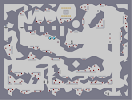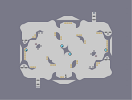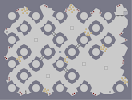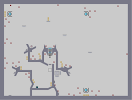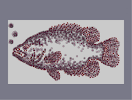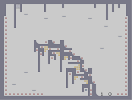1.5 Seconds Apart Apple Sauce Diablo Smoke Fish The Red Dance

## Comments

Pages: (2) [ 1 ] 2

*.*
5aved
5/5

im 150 to rate!

### wow

this is coolsometastic :P

### im pretty new

so i have only just seen this map it is amazing!!!! 5aved.

5/5

### Underrated

-_-
eh i mean superb lol
eh i mean superb lol

Suberb!

### u r

one hell of a NED artist...

### No life?

hehe, no life :P, anyways, i like hard wooden things :O lol kidding, Cool dead tree

### Wow!!!

How long did that take?!

### AWE

I've always thought your work was...
Dark.
But seriously guy, that's...Flippin' gooooooood!!!
5er.

very nice dude

### THATS AMAZING

awesome work dude.

### AWESOME!!!!!!!!

This map was spectacular! Amazing! It blew me away! Try Starry Night next time!!!!!
10000000000000000000000000000000000000000000/5
WOW WOW WOW WOW WOW WOW..........MONKEY!

### Thanks!

A N-Art DED? Feel free to submit and tell me when it's up ^_^

### congrats on 100

y'know, I should make you a dedication. Maybe the pathetic attmept at an N-art I've been working on?

### Za Best

That is soooooooooooo cool!!!
How long did it take 2 make...
It is soooooooooo good!!!
U have like 101 rates, and it is rated about 4.5/5

### Okayokay okay

I changed it to gausses now! :-P

I can't wait to see this goto 100 ratings, it will be my first.

### just to let you know

those arent lasers
they're gauss guns
lasers are the red drones...

but nice job

5aved

### Hmmm...

Its brilliant on this page but if u open it up fullsize, it looks nowhere ner as good

### OMG SO GOOD

IT'S AWSEM THE MONA LISA LIKE THIS WOULD BE SO COOL

### Why haven't I commented on this map yet?

Oh well, 5/5 and try the Mona Lisa next.

### WOOOOOOOOOOOOOOOOOOOOOOOOOOOOOOOOOOOOOOOOOOOW

AWSOME THE BEST NART EVER MADE(TOOK LIKE 5MIN TO LOAD
5/5)AND IT WOULDNT LET ME PLAY

Not lasers?

Fab.

### ....

Very.... interesting.

How long did this take you?

### you should make a forest

you know. with more trees. well, one is fine when it looks this amazing, but more trees would look, well... more amazing. like the next afternoon dragon or something of that sort.

5aved.

### COOL

Dude that is amazing!!!!!!!!!!!!!!!!!!!!!!!!!!

It's okay ;-)

oops double post

### OMG

its be-beautiful!
*starts weeping*

neways. it is such a step above anything that we've seen b4 (although afternoon dragon is spectacular). it is truely art. correct shapes adn beautiful shading. take it from one who is in honors art: this is a work of art. many would b lucky to get that even on paper. By far the best N art on Numa.
Congratulations
5aved

### OMG

its be-beautiful!
*starts weeping*

neways. it is such a step above anything that we've seen b4 (although afternoon dragon is spectacular). it is truely art. correct shapes adn beautiful shading. take it from one who is in honors art: this is a work of art. many would b lucky to get that even on paper. By far the best N art on Numa.
Congratulations
5aved

### Best N-Art Ever

No joke either. A lot better than Afternoon Dragon (N lends itself better to single color stuff)

### nice

lol you should put a ninja in there and start the game.
>.<

### It's BEAUTIFUL!!!

I have decided to use that picture of glory that you call "ART" Which should be called Beautiful painting as a wallpaper.
If i could, i'd give you 6/5, but i can't, so i'll have to stick to 5/5. *sigh*
Beautiful...